$$\newcommand{\id}{\mathrm{id}}$$ $$\newcommand{\Span}{\mathrm{span}}$$ $$\newcommand{\kernel}{\mathrm{null}\,}$$ $$\newcommand{\range}{\mathrm{range}\,}$$ $$\newcommand{\RealPart}{\mathrm{Re}}$$ $$\newcommand{\ImaginaryPart}{\mathrm{Im}}$$ $$\newcommand{\Argument}{\mathrm{Arg}}$$ $$\newcommand{\norm}{\| #1 \|}$$ $$\newcommand{\inner}{\langle #1, #2 \rangle}$$ $$\newcommand{\Span}{\mathrm{span}}$$

# 8.2: Parabolas

$$\newcommand{\vecs}{\overset { \rightharpoonup} {\mathbf{#1}} }$$ $$\newcommand{\vecd}{\overset{-\!-\!\rightharpoonup}{\vphantom{a}\smash {#1}}}$$$$\newcommand{\id}{\mathrm{id}}$$ $$\newcommand{\Span}{\mathrm{span}}$$ $$\newcommand{\kernel}{\mathrm{null}\,}$$ $$\newcommand{\range}{\mathrm{range}\,}$$ $$\newcommand{\RealPart}{\mathrm{Re}}$$ $$\newcommand{\ImaginaryPart}{\mathrm{Im}}$$ $$\newcommand{\Argument}{\mathrm{Arg}}$$ $$\newcommand{\norm}{\| #1 \|}$$ $$\newcommand{\inner}{\langle #1, #2 \rangle}$$ $$\newcommand{\Span}{\mathrm{span}}$$ $$\newcommand{\id}{\mathrm{id}}$$ $$\newcommand{\Span}{\mathrm{span}}$$ $$\newcommand{\kernel}{\mathrm{null}\,}$$ $$\newcommand{\range}{\mathrm{range}\,}$$ $$\newcommand{\RealPart}{\mathrm{Re}}$$ $$\newcommand{\ImaginaryPart}{\mathrm{Im}}$$ $$\newcommand{\Argument}{\mathrm{Arg}}$$ $$\newcommand{\norm}{\| #1 \|}$$ $$\newcommand{\inner}{\langle #1, #2 \rangle}$$ $$\newcommand{\Span}{\mathrm{span}}$$

##### Learning Objectives

By the end of this section, you will be able to:

• Graph vertical parabolas
• Graph horizontal parabolas
• Solve applications with parabolas

Before you get started, take this readiness quiz.

1. Graph: $$y=-3 x^{2}+12 x-12$$.
If you missed this problem, review Example 9.47.
2. Solve by completing the square: $$x^{2}-6 x+6=0$$.
If you missed this problem, review Example 9.12.
3. Write in standard form: $$y=3 x^{2}-6 x+5$$.
If you missed this problem, review Example 9.59.

## Graph Vertical Parabolas

The next conic section we will look at is a parabola. We define a parabola as all points in a plane that are the same distance from a fixed point and a fixed line. The fixed point is called the focus,and the fixed line is called the directrix of the parabola.

##### Definition $$\PageIndex{1}$$: Parabola, Focus, and Directrix

A parabola is all points in a plane that are the same distance from a fixed point and a fixed line. The fixed point is called the focus, and the fixed line is called the directrix of the parabola.

Previously, we learned to graph vertical parabolas from the general form or the standard form using properties. Those methods will also work here. We will summarize the properties here.

### Vertical Parabolas

General form

$$y=a x^{2}+b x+c$$

Standard Form

$$y=a(x-h)^{2}+k$$

Orientation $$a>0$$ up; $$a<0$$ down $$a>0$$ up; $$a<0$$ down
Axis of Symmetry $$x=-\frac{b}{2 a}$$ $$x=h$$
Vertex Substitute $$x=-\frac{b}{2 a}$$ and
solve for $$y .$$
$$(h, k)$$
$$y$$-intercept Let $$x=0$$ Let $$x=0$$
$$x$$-intercepts Let $$y=0$$ Let $$y=0$$
Table 11.2.1

The graphs show what the parabolas look like when they open up or down. Their position in relation to the $$x$$- or $$y$$-axis is merely an example.

To graph a parabola from these forms, we used the following steps.

##### Note

How to Graph Vertical Parabolas $$\left(y=a x^{2}+b x+c$$ or $$f(x)=a(x-h)^{2}+k\right)$$ using Properties.

Step 1: Determine whether the parabola opens upward or downward.

Step 2. Find the axis of symmetry.

Step 3. Find the vertex.

Step 4. Find the $$y$$-intercept. Find the point symmetric to the $$y$$-intercept across the axis of symmetry.

Step 5. Find the $$x$$-intercepts.

Step 6. Graph the parabola.

The next example reviews the method of graphing a parabola from the general form of its equation.

##### Example $$\PageIndex{1}$$

Graph $$y=-x^{2}+6 x-8$$ by using properties.

Solution:Since $$a$$ is $$-1$$, the parabola opens downward.To find the axis of symmetry, find $$x=-\frac{b}{2 a}$$.The axis of symmetry is $$x=3$$.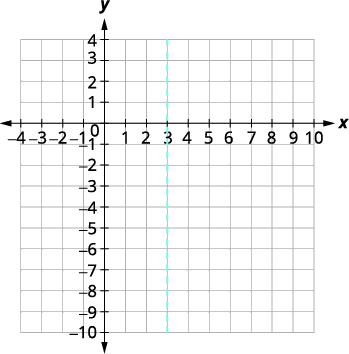The vertex is on the line $$x=3$$.Let $$x=3$$.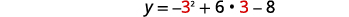The vertex is $$(3,1)$$.The $$y$$-intercept occurs when $$x=0$$.Substitute $$x=0$$.Simplify.The $$y$$-intercept is $$(0,-8)$$. The point $$(0,−8)$$ is three units to the left of the line of symmetry. The point three units to the right of the line of symmetry is $$(6,−8)$$. Point symmetric to the $$y$$-intercept is $$(6,−8)$$.The $$x$$-intercept occurs when $$y=0$$.Let $$y=0$$.Factor the GCF.Factor the trinomial.Solve for $$x$$.The $$x$$-intercepts are $$(4,0),(2,0)$$. Graph the parabola.##### Exercise $$\PageIndex{1}$$

Graph $$y=-x^{2}+5 x-6$$ by using properties.

##### Exercise $$\PageIndex{2}$$

Graph $$y=-x^{2}+8 x-12$$ by using properties.

The next example reviews the method of graphing a parabola from the standard form of its equation, $$y=a(x-h)^{2}+k$$.

##### Example $$\PageIndex{2}$$

Write $$y=3 x^{2}-6 x+5$$ in standard form and then use properties of standard form to graph the equation.

Solution:

 Rewrite the function in $$y=a(x-h)^{2}+k$$ form by completing the square. $$y=3(x-1)^{2}+2$$ Identify the constants $$a, h, k$$. $$a=3, h=1, k=2$$ Since $$a=2$$, the parabola opens upward.The axis of symmetry is $$x=h$$. The axis of symmetry is $$x=1$$. The vertex is $$(h,k)$$. The vertex is $$(1,2)$$. Find the $$y$$-intercept by substituting $$x=0$$, $$y=3(x-1)^{2}+2$$ $$y=3 \cdot 0^{2}-6 \cdot 0+5$$ $$y=5$$ $$y$$-intercept $$(0,5)$$ Find the point symmetric to $$(0,5)$$ across the axis of symmetry. $$(2,5)$$ Find the $$x$$-intercepts. The square root of a negative number tells us the solutions are complex numbers. So there are no $$x$$-intercepts. Graph the parabola.##### Exercise $$\PageIndex{3}$$
1. Write $$y=2 x^{2}+4 x+5$$ in standard form and
2. use properties of standard form to graph the equation.
1. $$y=2(x+1)^{2}+3$$
##### Exercise $$\PageIndex{4}$$
1. Write $$y=-2 x^{2}+8 x-7$$ in standard form and
2. use properties of standard form to graph the equation.
1. $$y=-2(x-2)^{2}+1$$

## Graph Horizontal Parabolas

Our work so far has only dealt with parabolas that open up or down. We are now going to look at horizontal parabolas. These parabolas open either to the left or to the right. If we interchange the $$x$$ and $$y$$ in our previous equations for parabolas, we get the equations for the parabolas that open to the left or to the right.

### Horizontal Parabolas

General form

$$x=a y^{2}+b y+c$$

Standard form

$$x=a(y-k)^{2}+h$$

Orientation $$a>0$$ right; $$a<0$$ left $$a>0$$ right; $$a<0$$ left
Axis of Symmetry $$y=-\frac{b}{2 a}$$ $$y=k$$
Vertex Substitute $$y=-\frac{b}{2 a}$$ and
solve for $$x .$$
$$(h, k)$$
$$x$$-intercepts Let $$x=0$$ Let $$x=0$$
$$y$$-intercept Let $$y=0$$ Let $$y=0$$
Table 11.2.4

The graphs show what the parabolas look like when they to the left or to the right. Their position in relation to the $$x$$- or $$y$$-axis is merely an example.

Looking at these parabolas, do their graphs represent a function? Since both graphs would fail the vertical line test, they do not represent a function.

To graph a parabola that opens to the left or to the right is basically the same as what we did for parabolas that open up or down, with the reversal of the $$x$$ and $$y$$ variables.

##### Howto: Graph Horizontal Parabolas $$\left(y=a x^{2}+b x+c$$ or $$f(x)=a(x-h)^{2}+k\right)$$ using Properties
• Step 1: Determine whether the parabola opens to the left or to the right.
• Step 2: Find the axis of symmetry.
• Step 3: Find the vertex.
• Step 4: Find the $$x$$-intercept. Find the point symmetric to the $$x$$-intercept across the axis of symmetry.
• Step 5: Find the $$y$$-intercepts.
• Step 6: Graph the parabola.
##### Example $$\PageIndex{3}$$

Graph $$x=2 y^{2}$$ by using properties.

Solution:Since $$a=2$$, the parabola opens to the right.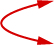To find the axis of symmetry, find $$y=-\frac{b}{2 a}$$The axis of symmetry is $$y=0$$. The vertex is on the line $$y=0$$.Let $$y=0$$.The vertex is $$(0,0)$$.

Since the vertex is $$(0,0)$$, both the $$x$$- and $$y$$-intercepts are the point $$(0,0)$$. To graph the parabola we need more points. In this case it is easiest to choose values of $$y$$.

We also plot the points symmetric to $$(2,1)$$ and $$(8,2)$$ across the $$y$$-axis, the points $$(2,−1),(8,−2)$$.

Graph the parabola.

##### Exercise $$\PageIndex{5}$$

Graph $$x=y^{2}$$ by using properties.

##### Exercise $$\PageIndex{6}$$

Graph $$x=-y^{2}$$ by using properties.

In the next example, the vertex is not the origin.

##### Example $$\PageIndex{4}$$

Graph $$x=-y^{2}+2 y+8$$ by using properties.

Solution:Since $$a=-1$$, the parabola opens to the left.To find the axis of symmetry, find $$y=-\frac{b}{2 a}$$The axis of symmetry is $$y=1$$. The vertex is on the line $$y=1$$.Let $$y=1$$.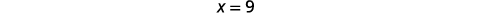The vertex is $$(9,1)$$. The $$x$$-intercept occurs when $$y=0$$.The $$x$$-intercept is $$(8,0)$$. The point $$(8,0)$$ is one unit below the line of symmetry. The symmetric point one unit above the line of symmetry is $$(8,2)$$ Symmetric point is $$(8,2)$$. The $$y$$-intercept occurs when $$x=0$$.Substitute $$x=0$$.Solve.The $$y$$ -intercepts are $$(0,4)$$ and $$(0,-2)$$. Connect the points to graph the parabola.##### Exercise $$\PageIndex{7}$$

Graph $$x=-y^{2}-4 y+12$$ by using properties.

##### Exercise $$\PageIndex{8}$$

Graph $$x=-y^{2}+2 y-3$$ by using properties.

In Table 11.2.4, we see the relationship between the equation in standard form and the properties of the parabola. The How To box lists the steps for graphing a parabola in the standard form $$x=a(y-k)^{2}+h$$. We will use this procedure in the next example.

##### Example $$\PageIndex{5}$$

Graph $$x=2(y-2)^{2}+1$$ using properties.

Solution:Identify the constants $$a, h, k$$. $$a=2, h=1, k=2$$ Since $$a=2$$, the parabola opens to the right.The axis of symmetry is $$y=k$$. The axis of symmetry is $$y=2$$. The vertex is $$(h,k)$$. The vertex is $$(1,2)$$. Find the $$x$$-intercept by substituting $$y=0$$. $$x=2(y-2)^{2}+1$$ $$x=2(0-2)^{2}+1$$ $$x=9$$ The $$x$$-intercept is $$(9,0)$$. Find the point symmetric to $$(9,0)$$ across the axis of symmetry. $$(9,4)$$ Find the $$y$$-intercepts. Let $$x=0$$. A square cannot be negative, so there is no real solution. So there are no $$y$$-intercepts. Graph the parabola.##### Exercise $$\PageIndex{9}$$

Graph $$x=3(y-1)^{2}+2$$ using properties.

##### Exercise $$\PageIndex{10}$$

Graph $$x=2(y-3)^{2}+2$$ using properties.

In the next example, we notice the a is negative and so the parabola opens to the left.

##### Example $$\PageIndex{6}$$

Graph $$x=-4(y+1)^{2}+4$$ using properties.

Solution:Identify the constants $$a, h, k$$. $$a=-4, h=4, k=-1$$ Since $$a=-4$$, the parabola opens to the left.The axis of symmetry is $$y=k$$. The axis of symmetry is $$y=-1$$. The vertex is $$(h,k)$$. The vertex is $$(4,-1)$$. Find the $$x$$-intercept by substituting $$y=0$$. $$x=-4(y+1)^{2}+4$$ $$x=-4(0+1)^{2}+4$$ $$x=0$$ The $$x$$-intercept is $$(0,0)$$. Find the point symmetric to $$(0,0)$$ across the axis of symmetry. $$(0,-2)$$ Find the $$y$$-intercepts. $$x=-4(y+1)^{2}+4$$ Let $$x=0$$. $$y=-1+1 \quad y=-1-1$$ $$y=0 \quad\quad y=-2$$ The $$y$$-intercepts are $$(0,0)$$ and $$(0,-2)$$. Graph the parabola.##### Exercise $$\PageIndex{11}$$

Graph $$x=-4(y+2)^{2}+4$$ using properties.

##### Exercise $$\PageIndex{12}$$

Graph $$x=-2(y+3)^{2}+2$$ using properties.

The next example requires that we first put the equation in standard form and then use the properties.

##### Example $$\PageIndex{7}$$

Write $$x=2 y^{2}+12 y+17$$ in standard form and then use the properties of the standard form to graph the equation.

Solution:Rewrite the function in $$x=a(y-k)^{2}+h$$ form by completing the square.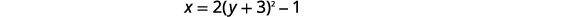Identify the constants $$a, h, k$$. $$a=2, h=-1, k=-3$$ Since $$a=2$$, the parabola opens to the right.The axis of symmetry is $$y=k$$. The axis of symmetry is $$y=-3$$. The vertex is $$(h,k)$$. The vertex is $$(-1,-3)$$. Find the $$x$$-intercept by substituting $$y=0$$. $$x=2(y+3)^{2}-1$$ $$x=2(0+3)^{2}-1$$ $$x=17$$ The $$x$$-intercept is $$(17,0)$$. Find the point symmetric to $$(17,0)$$ across the axis of symmetry. $$(17,-6)$$ Find the $$y$$-intercepts. Let $$x=0$$. $$y=-3+\frac{\sqrt{2}}{2} \quad y=-3-\frac{\sqrt{2}}{2}$$ $$y \approx-2.3 \quad y \approx-3.7$$ The $$y$$-intercepts are $$\left(0,-3+\frac{\sqrt{2}}{2}\right),\left(0,-3-\frac{\sqrt{2}}{2}\right)$$. Graph the parabola.##### Exercise $$\PageIndex{13}$$
1. Write $$x=3 y^{2}+6 y+7$$ in standard form and
2. Use properties of the standard form to graph the equation.
1. $$x=3(y+1)^{2}+4$$
##### Exercise $$\PageIndex{14}$$
1. Write $$x=-4 y^{2}-16 y-12$$ in standard form and
2. Use properties of the standard form to graph the equation.
1. $$x=-4(y+2)^{2}+4$$

## Solve Applications with Parabolas

Many architectural designs incorporate parabolas. It is not uncommon for bridges to be constructed using parabolas as we will see in the next example.

##### Example $$\PageIndex{8}$$

Find the equation of the parabolic arch formed in the foundation of the bridge shown. Write the equation in standard form.

Solution:

We will first set up a coordinate system and draw the parabola. The graph will give us the information we need to write the equation of the graph in the standard form $$y=a(x-h)^{2}+k$$.

 Let the lower left side of the bridge be the origin of the coordinate grid at the point $$(0,0)$$. Since the base is $$20$$ feet wide the point $$(20,0)$$ represents the lower right side. The bridge is 10 feet high at the highest point. The highest point is the vertex of the parabola so the $$y$$-coordinate of the vertex will be $$10$$. Since the bridge is symmetric, the vertex must fall halfway between the left most point, $$(0,0)$$, and the rightmost point $$(20,0)$$. From this we know that the $$x$$-coordinate of the vertex will also be $$10$$.Identify the vertex, $$(h,k)$$. $$(h, k)=(10,10)$$ $$h=10, \quad k=10$$ Substitute the values into the standard form. The value of $$a$$ is still unknown. To find the value of $$a$$ use one of the other points on the parabola. \begin{aligned} y &=a(x-h)^{2}+k \\ y &=a(x-10)^{2}+10 \\(x, y) &=(0,0) \end{aligned} Substitute the values of the other point into the equation. $$y=a(x-10)^{2}+10$$ $$0=a(0-10)^{2}+10$$ Solve for $$a$$. $$y=a(x-10)^{2}+10$$ Substitute the value for $$a$$ into the equation. $$y=-\frac{1}{10}(x-10)^{2}+10$$
##### Exercise $$\PageIndex{15}$$

Find the equation of the parabolic arch formed in the foundation of the bridge shown. Write the equation in standard form.

$$y=-\frac{1}{20}(x-20)^{2}+20$$

##### Exercise $$\PageIndex{16}$$

Find the equation of the parabolic arch formed in the foundation of the bridge shown. Write the equation in standard form.

$$y=-\frac{1}{5} x^{2}+2 x y=-\frac{1}{5}(x-5)^{2}+5$$

Access these online resources for additional instructions and practice with quadratic functions and parabolas.

• Introduction to Conics and Graphing Horizontal Parabolas

## Key Concepts

• Parabola: A parabola is all points in a plane that are the same distance from a fixed point and a fixed line. The fixed point is called the focus, and the fixed line is called the directrix of the parabola.

### Vertical Parabolas

General form

$$y=a x^{2}+b x+c$$

Standard Form

$$y=a(x-h)^{2}+k$$

Orientation $$a>0$$ up; $$a<0$$ down $$a>0$$ up; $$a<0$$ down
Axis of Symmetry $$x=-\frac{b}{2 a}$$ $$x=h$$
Vertex Substitute $$x=-\frac{b}{2 a}$$ and
solve for $$y .$$
$$(h, k)$$
$$y$$-intercept Let $$x=0$$ Let $$x=0$$
$$x$$-intercepts Let $$y=0$$ Let $$y=0$$
Table 11.2.1
• How to graph vertical parabolas $$\left(y=a x^{2}+b x+c$$ or $$f(x)=a(x-h)^{2}+k\right)$$ using properties.
1. Determine whether the parabola opens upward or downward.
2. Find the axis of symmetry.
3. Find the vertex.
4. Find the $$y$$-intercept. Find the point symmetric to the $$y$$-intercept across the axis of symmetry.
5. Find the $$x$$-intercepts.
6. Graph the parabola.

### Horizontal Parabolas

General form

$$x=a y^{2}+b y+c$$

Standard form

$$x=a(y-k)^{2}+h$$

Orientation $$a>0$$ right; $$a<0$$ left $$a>0$$ right; $$a<0$$ left
Axis of Symmetry $$y=-\frac{b}{2 a}$$ $$y=k$$
Vertex Substitute $$y=-\frac{b}{2 a}$$ and
solve for $$x .$$
$$(h, k)$$
$$x$$-intercepts Let $$x=0$$ Let $$x=0$$
$$y$$-intercept Let $$y=0$$ Let $$y=0$$
Table 11.2.4
• How to graph horizontal parabolas $$\left(x=a y^{2}+b y+c$$ or $$x=a(y-k)^{2}+h\right)$$ using properties.
4. Find the $$x$$-intercept. Find the point symmetric to the $$x$$-intercept across the axis of symmetry.
5. Find the $$y$$-intercepts.